# Lists CS 5010 Program Design Paradigms Bootcamp Lesson

• Slides: 27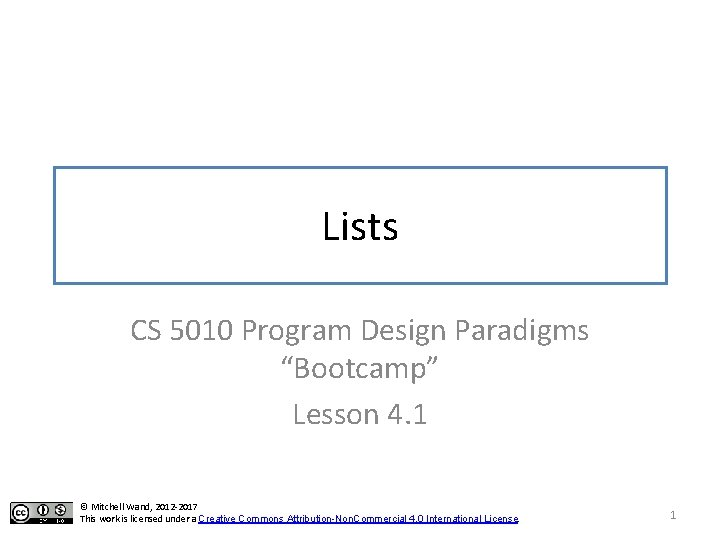Module 04 Basic Principles Tools and Techniques Object-Oriented Programming Designing Data Computing with Lists Interfaces and Classes Designing Functions Computing with Trees and Graphs Inheritance Designing Systems Computing with Higher-Order Functions Objects with Mutable State Designing with Invariants Efficiency, Part 2 Thinking about Efficiency 2Module Introduction • This week, we will learn about list data, which is a natural representation for sequences. • We will learn about – the arithmetic of lists – the observer template for list data – lists of structures 3Learning Objectives for Lesson 4. 1 At the end of this lesson, you should be able to: • Write down a data definition for information represented as a list • Notate lists using constructor, list, and write notations. • Explain how lists are represented as singly-linked data structures, and how cons, first, and rest work on these structures • Calculate with the basic operations on lists: cons, first, and rest. So let’s get started. . . 4Sequence Information • a phone book, which is a sequence of listings • a presentation, which is a sequence of slides • In Racket, these can be represented as lists. – for some applications, there are more efficient representations, but we’ll start with lists. 5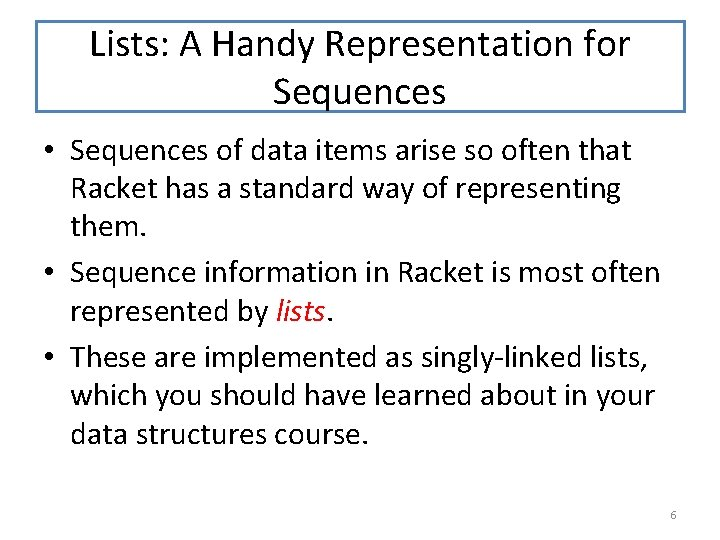Lists: A Handy Representation for Sequences • Sequences of data items arise so often that Racket has a standard way of representing them. • Sequence information in Racket is most often represented by lists. • These are implemented as singly-linked lists, which you should have learned about in your data structures course. 6Example Data Definition for Sequence Data There are many ways to represent a sequence of numbers. Here we’ve chosen to represent a sequence as a singly-linked list. ; ; DATA DEFINITION: ; ; ; ; ; A Number. Seq is represented as a list of Number. CONSTRUCTOR TEMPLATES: There are two ways to build a Number. Seq, so empty sequence we-havethe two constructor templates. We’ll deal with the observer template in the (cons n ns) next lesson. WHERE: n is a Number -- the first number in the sequence ns is a Number. Seq -- the rest of the numbers in the sequence We must give an interpretation for each possible Number. Seq. empty and cons are built into Racket. We don’t need any define-structures for them 7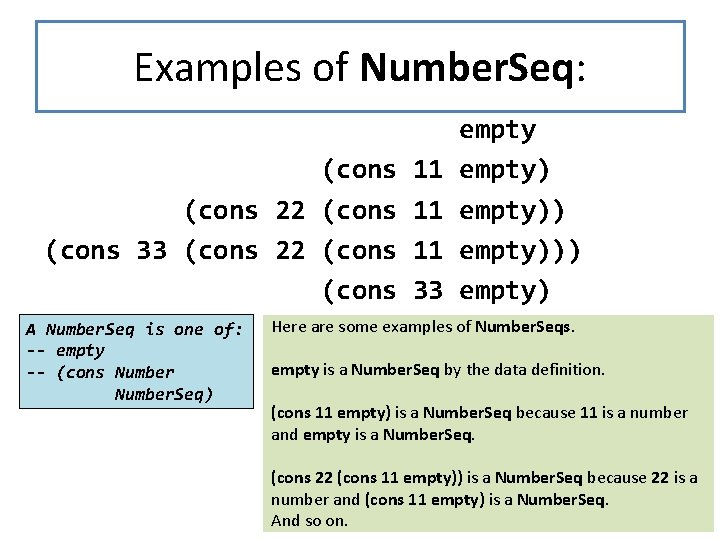Examples of Number. Seq: (cons 22 (cons 33 (cons 22 (cons A Number. Seq is one of: -- empty -- (cons Number. Seq) 11 11 11 33 empty))) empty) Here are some examples of Number. Seqs. empty is a Number. Seq by the data definition. (cons 11 empty) is a Number. Seq because 11 is a number and empty is a Number. Seq. (cons 22 (cons 11 empty)) is a Number. Seq because 22 is a number and (cons 11 empty) is a Number. Seq. 8 And so on.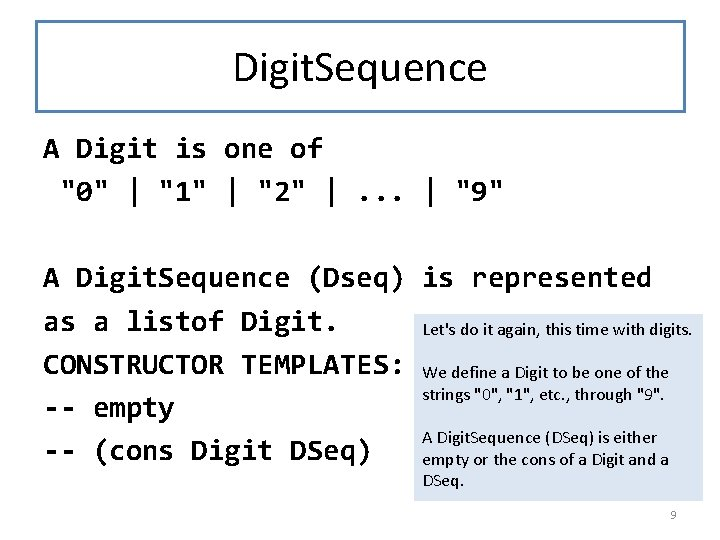Digit. Sequence A Digit is one of "0" | "1" | "2" |. . . | "9" A Digit. Sequence (Dseq) is represented as a listof Digit. Let's do it again, this time with digits. CONSTRUCTOR TEMPLATES: We define a Digit to be one of the strings "0", "1", etc. , through "9". -- empty A Digit. Sequence (DSeq) is either -- (cons Digit DSeq) empty or the cons of a Digit and a DSeq. 9Examples of DSeqs empty (cons "3" empty) (cons "2" (cons "3" empty)) (cons "4" (cons "2" (cons "3" empty))) • These are not DSeqs: (cons 4 (cons "2" (cons "3" empty))) (cons "3" empty) (cons "2" (cons "3" empty))) A Digit. Sequence (DSeq) is one of: -- empty -- (cons Digit DSeq) Can you explain why each of the first 4 examples are DSeq’s, according to the data definition? Can you explain why the last two are 10 not Dseq’s?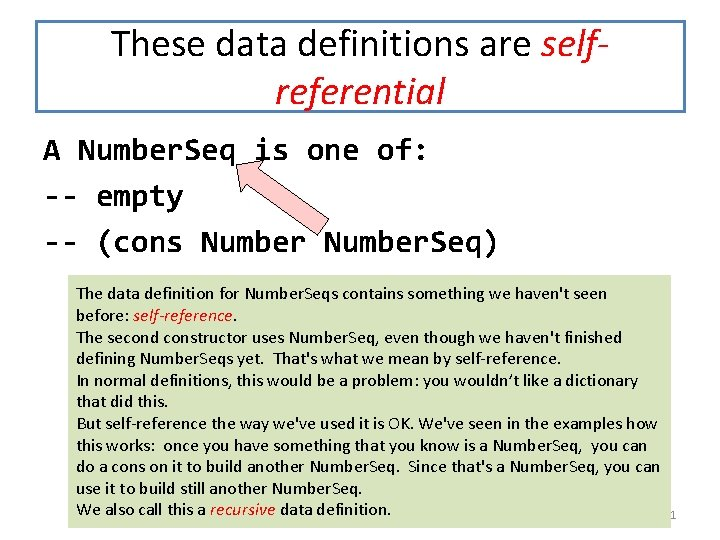These data definitions are selfreferential A Number. Seq is one of: -- empty -- (cons Number. Seq) The data definition for Number. Seqs contains something we haven't seen before: self-reference. The second constructor uses Number. Seq, even though we haven't finished defining Number. Seqs yet. That's what we mean by self-reference. In normal definitions, this would be a problem: you wouldn’t like a dictionary that did this. But self-reference the way we've used it is OK. We've seen in the examples how this works: once you have something that you know is a Number. Seq, you can do a cons on it to build another Number. Seq. Since that's a Number. Seq, you can use it to build still another Number. Seq. We also call this a recursive data definition. 11This one is self-referential, too A Digit is one of "0" | "1" | "2" |. . . | "9" A Digit. Sequence (DSeq) is one of: -- empty -- (cons Digit DSeq) 12How Lists Represent Sequences • If X is some data definition, we define a list of X's as either empty or the cons of an X and a list of X's. • So a list of sardines is either empty or the cons of a sardine and a list of sardines. • The interpretation is always "a sequence of X's". – empty represents a sequence with no elements – (cons x lst) represents a sequence whose first element is x and whose other elements are represented by lst. • If we had some information that we wanted to represent as a list of X's (say a list of people), we would have to specify the order in which the X's appear (say "in increasing order of height"), or else say “in any order. ” 13The constructor template for list data CONSTRUCTOR TEMPLATES for XList -- empty interp: a sequence of X's with no elements -- (cons X XList) interp: (cons x xs) represents a sequence of X's whose first element is x and whose other elements are represented by xs. We’ve written XList here, but there is no such thing as an XList. There are only Number. Lists, or Digit. Lists, or Sardine. Lists. We’ll write XList when we mean a list of X’s for some X, but we don’t care which it is. We use xs (pronounced "ex's") as the plural of "x". 14Collection Information • Sometimes we are interested in representing a set, which may not have a built-in notion of order – a space-invaders game with many invaders – a book-store inventory with many books • You can use lists to represent these as well. – You will need to specify the order (or lack thereof) – This will be part of the data definition 15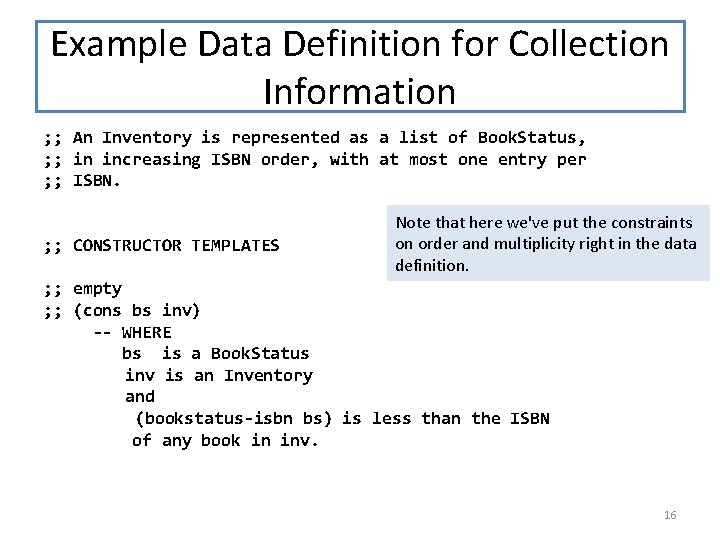Example Data Definition for Collection Information ; ; An Inventory is represented as a list of Book. Status, ; ; in increasing ISBN order, with at most one entry per ; ; ISBN. ; ; CONSTRUCTOR TEMPLATES Note that here we've put the constraints on order and multiplicity right in the data definition. ; ; empty ; ; (cons bs inv) -- WHERE bs is a Book. Status inv is an Inventory and (bookstatus-isbn bs) is less than the ISBN of any book in inv. 16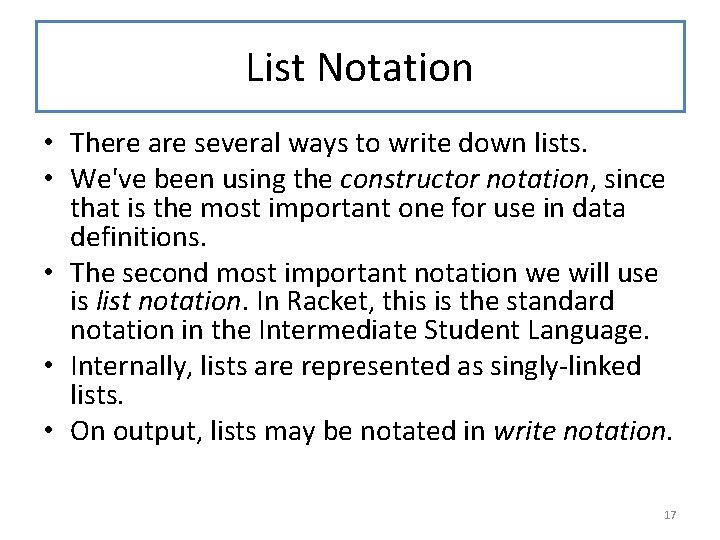List Notation • There are several ways to write down lists. • We've been using the constructor notation, since that is the most important one for use in data definitions. • The second most important notation we will use is list notation. In Racket, this is the standard notation in the Intermediate Student Language. • Internally, lists are represented as singly-linked lists. • On output, lists may be notated in write notation. 17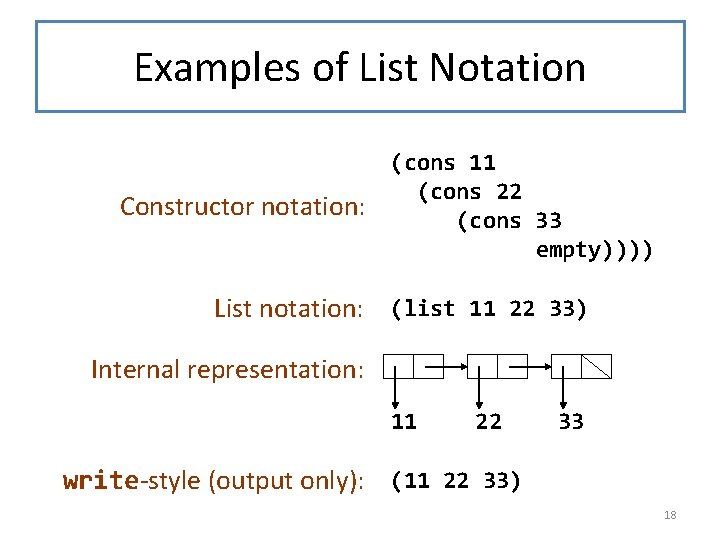Examples of List Notation Constructor notation: (cons 11 (cons 22 (cons 33 empty)))) List notation: (list 11 22 33) Internal representation: 11 22 33 write-style (output only): (11 22 33) 18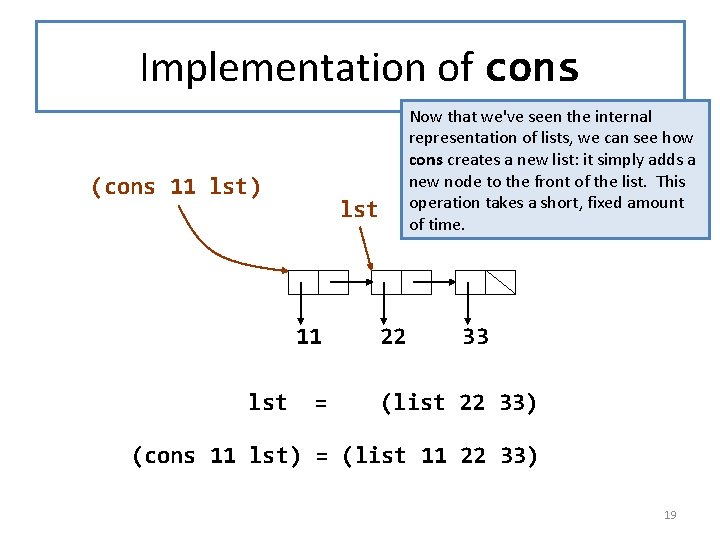Implementation of cons (cons 11 lst) lst 11 lst Now that we've seen the internal representation of lists, we can see how cons creates a new list: it simply adds a new node to the front of the list. This operation takes a short, fixed amount of time. = 22 33 (list 22 33) (cons 11 lst) = (list 11 22 33) 19Operations on Lists empty? : XList -> Boolean Given a list of X's, returns true iff the list is empty Racket provides 3 functions for inspecting lists and taking them apart. These are empty? , first, and rest. The predicate empty? returns true if and only if the list is empty. 20Operations on Lists first : XList -> X GIVEN: a non-empty list of X’s RETURNS: the first element in the list. When we write down the template for lists, we will see that when we call first, its argument will always be nonempty. 21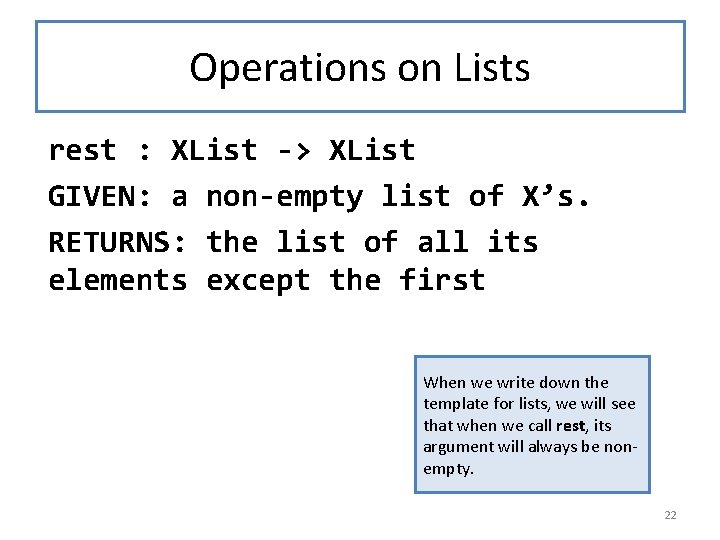Operations on Lists rest : XList -> XList GIVEN: a non-empty list of X’s. RETURNS: the list of all its elements except the first When we write down the template for lists, we will see that when we call rest, its argument will always be nonempty. 22Examples (empty? empty) = true (empty? (cons 11 empty)) = false (empty? (cons 22 (cons 11 empty))) = false (first (cons 11 empty)) = 11 (rest (cons 11 empty)) = empty (first (cons 22 (cons 11 empty))) = 22 (rest (cons 22 (cons 11 empty))) = (cons 11 empty) (first empty) (rest empty) Error! (Precondition failed) 23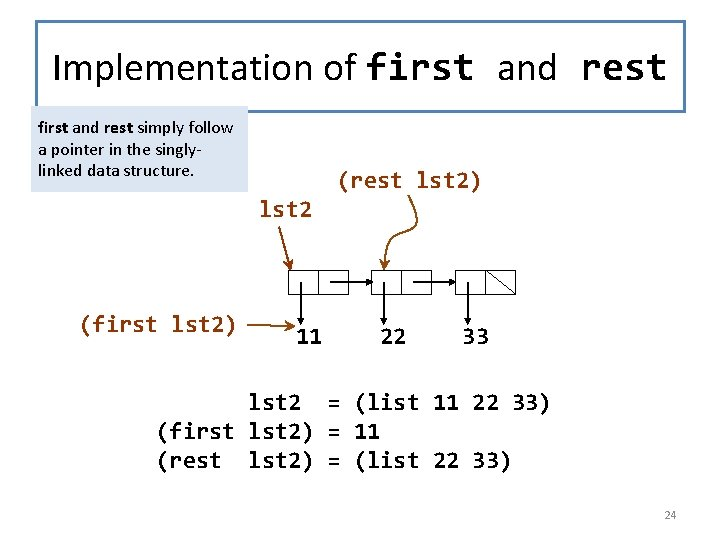Implementation of first and rest simply follow a pointer in the singlylinked data structure. (rest lst 2) lst 2 (first lst 2) 11 22 33 lst 2 = (list 11 22 33) (first lst 2) = 11 (rest lst 2) = (list 22 33) 24Properties of cons, first, and rest (first (cons v l)) = v (rest (cons v l)) = l If l is non-empty, then (cons (first l) (rest l)) = l Here are some useful facts about first, rest, and cons. Can you see why they are true? These facts tell us that if we want to build a list whose first is x and whose rest is lst, we can do this by writing (cons x lst). 25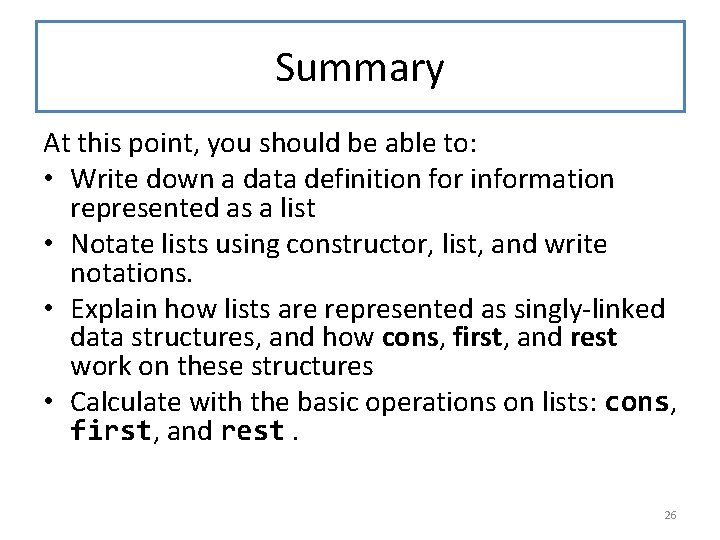Summary At this point, you should be able to: • Write down a data definition for information represented as a list • Notate lists using constructor, list, and write notations. • Explain how lists are represented as singly-linked data structures, and how cons, first, and rest work on these structures • Calculate with the basic operations on lists: cons, first, and rest. 26Next Steps • If you have questions about this lesson, ask them on the Discussion Board • Do Guided Practices 4. 1 and 4. 2 • Go on to the next lesson 27